### Eurocode 2 model for concrete creep and shrinkage - EC2CreepMat

Implementation of aging viscoelastic model for concrete creep according to Eurocode 2 for concrete structures The model parameters are summarized in Tab. 34.

According to EC2, the compliance function is defined using the creep coefficient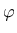as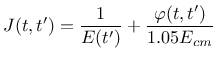(92)

whereis the mean elastic modulus at the age of 28 days and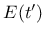is the elastic modulus at the age of loading,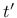.

Current implementation supports only linear creep which is valid only for stresses below 0.45 of the characteristic compressive strength at the time of loading.

The elastic modulus at age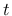(in days) is defined as(93)

whereis a cement-type dependent constant (0.2 for class R, 0.25 for class N and finally 0.38 for type S), and the mean secant elastic modulus at 28 days can be estimated from the mean compressive strength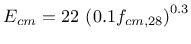(94)

where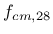is in MPa and the resulting modulus is in GPa.

The creep coefficient is given by the expression from Annex B of the standard.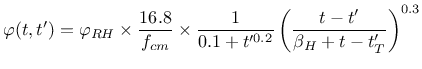(95)

with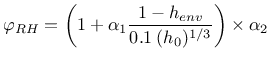(96)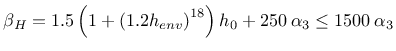(97)

and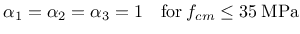(98)

else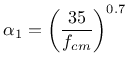(99)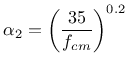(100)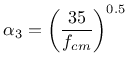(101)

and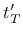is the temperature-adusted age according to B.9 in the code. It is computed automatically if string temperatureDependent appears in the input record.

The shrinkage deformation is additively split into two parts: drying shrinkage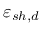and autogenous shrinkage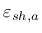. Drying shrinkage strain at timeis computed from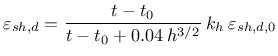(102)

where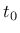is the duration of curing,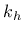is a thickness-dependent parameter and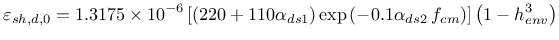(103)

with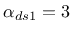for cement class S, 4 for class N, and 6 for class R, and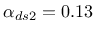for cement class S, 0.12 for class N, and 0.11 for class R.

Autogenous shrinkage strain can be comptued as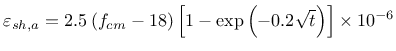(104)

Table 34: EC2Creep material model - summary.
 Description EC2CreepMat model for concrete creep and shrinkage Record Format EC2CreepMat n(rn) # [ begOfTimeOfInterest(rn) #] [ endOfTimeOfInterest(rn) #] relMatAge(rn) # [ timeFactor(rn) #] stiffnessFactor(rn) # [ tAlpha(rn) #] fcm28(rn) # t0(rn) # cemType(in) # [ henv(rn) #] h0(rn) # shType(in) # [ spectrum ] [ temperatureDependent ] Parameters - num material model number - n Poisson's ratio - begOfTimeOfInterest determines the shortest time which is reasonably well captured by the approximated compliance function (default value is 0.1); the units are the time units of the analysis - endOfTimeOfInterest determines the longest time which is reasonably well captured by the approximated compliance function (if not provided it is read from the engineering model); the units are the time units of the analysis - relMatAge time shift used to specify the age of material on the begging of the analysis, the meaning is the material age at time t = 0; - timeFactor scaling factor transforming the actual time into appropriate units needed by the formulae of the eurocode. For analysis in days timeFactor = 1, for analysis in seconds timeFactor = 86,400. - stiffnessFactor scaling factor transforming predicted stiffness into appropriate units of the analysis, for analysis in MPa stiffnessFactor = 1.e6 (default), for Pa stiffnessFactor = 1 - fcm28 mean compressive strength measured on cylinders at the age of 28 days in MPa - t0 duration of curing [day] (this is relevant only for drying shrinkage, not for creep) - cemType type of cement, 1 = class R, 2 = class N, 3 = class S - henv ambient relative humidity expressed as decimal - h0 effective member thickness in [mm] calculated according to EC2 as 2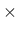A/u where A is the cross-section are and u is the cross-section perimeter exposed to drying - shType shrinkage type; 0 = no shrinkage, 1 = both drying and autogenous shrinkage, 2 = drying shrinkage only, 3 = autogenous shrinkage only - spectrum this string switches on evaluation of the moduli of the aging Kelvin chain using the retardation spectrum of the compliance function, otherwise (default option) the least-squares method is used - temperatureDependent turns on the influence of temperature on concrete maturity (equivalent age concept) by default this option is not activated. Supported modes 3dMat, PlaneStress, PlaneStrain, 1dMat, 2dPlateLayer,2dBeamLayer, 3dShellLayer

Borek Patzak
2019-03-19